Earth Sciences
Volume 4, Issue 5, October 2015, Pages: 188-192

Application of Chaotic Time-Series Optimization Algorithm in Solving the Parameters of Leakage Aquifer

Zhang Xiao-zhou1, 2, Lu Yu-dong1, 2, Guo Jian-qing1, 2

1Environment Science and Engineering Institute, Chang'an University, Xi'an, China

2Key Laboratory of Subsurface Hydrology and Ecology in Arid Areas, Ministry of Education, Xi'an, China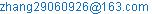(Zhang Xiao-zhou)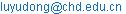(Lu Yu-dong)

Zhang Xiao-zhou, Lu Yu-dong, Guo Jian-qing. Application of Chaotic Time-Series Optimization Algorithm in Solving the Parameters of Leakage Aquifer. Earth Sciences. Vol. 4, No. 5, 2015, pp. 188-192. doi: 10.11648/j.earth.20150405.14

Abstract: Applied the chaotic time-series optimization algorithm to solve hydrogeological parameters through analysis the pumping test data of the first type leakage aquifer, then explored the influence of the initial value of aquifer parameters and other factors on the convergence and results of the algorithm. The results shown that: chaotic time-series optimization algorithm could be effectively applied to the calculation problem of aquifer parameters; the initial value of the coefficient of storage and conductivity don’t have too much obvious effect on the search and results of the algorithm; The upper limit of algorithm had no obvious effect on the search ability but reduce the accuracy of results. Compared with other methods, the chaotic optimization method had such advantages as simple in principle of algorithm, easy to make program and to conduct, and the precision of aquifer parameters calculated was not affected by artificial subjective factors.

Keywords: Chaotic Time-Series Optimization Algorithm, Leakage System, Leakage Factor

1. Introduction

In the process of determining the hydrogeological parameters of aquifer, the standard curve comparison method, inflection point method, tangent method  had got a very good application, but in practice, because of the difference between the human and the mapping, the results can be differ in thousands of ways. Against this, WANG Yuan-hui , SHI Zhi-yuan , LUO Jun , et al., applied the chaos particle swarm algorithm, genetic algorithm and bee colony algorithm to analysis of pumping test data and determine the parameters of aquifer respectively, and got a good result. The most successful was the utilized of chaotic time-series optimization algorithm to on Theis well flow. In this paper, the algorithm of chaos time-series optimization in determining the parameters of aquifer was proposed, the results were reliable contrasted with the results of Theis wiring method  and Sushil K.s. method. For the chaotic time-series optimization algorithm was a direct random search, and the characteristics of the objective function itself was less, so it had a wide range of applications . In addition, because the search process of the chaotic time-series optimization algorithm was accomplished by writing program, involved little human disturbance factors, so the results of the calculation were very high objectivity, stability and timeliness, compared with the traditional wiring method, it had a great advantage.

However, literature  only analyze the application in single-well without leakage, for the application in multi-well condition with leakage aquifer was not described. For this reason, the author analyze the first type leakage aquifer of multi-well pumping test data and determined the aquifer parameters, to study the further application of chaos time-series optimization algorithm in determining the parameters of aquifer.

2. Ideas of Algorithm

Based on the chaotic dynamics the search process  can be divided into the following two basic processes:

First, a specific iteration method was determined to obtain an ergodic orbit, which could be used to investigate the whole solution space. Search process of the algorithm was carried out in this space, the process would be ended when it meeting the certain conditions, and we considered the optimal solution in this search process was close to the optimal solution of the problem, and this optimal solution was used as the starting point of the second time search. This process was called a rough search process. Then, a small amplitude perturbation was added to the results obtained from the above to conduct further search in the local area, finally reached the termination criterion of the algorithm. This process was called the fine search process.

Based on the above ideas, Li Bing et al. (1997) used the carrier method transformed the chaotic variables generated by the Logistic map into the optimization variables, at the same time, the ergodicity range of the chaotic motion was converted to the optimization variable . Next, we used the chaotic variable to carry on the twice searchers of the aquifer parameters. Its concrete steps are as follows:

Step 1: Let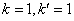, for the logistic mapping, the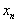in the equation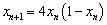were endowed with i-initial values with small differences, therefor we can got i-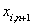with different trajectories. There, i is number of parameters to be found;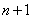is length of chaotic time-series.

Step 2:Through the equation of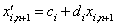, the chaos variablewas transformed into the optimization variables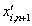by carrier method. There, the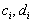is a constant, these two parameters are used to scale the optimization variables.

Step 3: Using the first iteration of the chaotic variable, let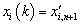, to calculate the corresponding function optimization value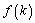. Let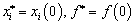. If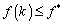then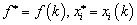; else, abandoned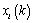. Let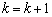.

Step 4: If the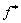always maintain a certain value or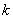is greater than the certain value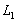(the rough search times) after several searches in step 3, we considered the rough search stage was end, the algorithm can enter the fine search stage, else, return to step 3.

Step 5: After the step 4 the second carrier was executed in accordance with the equation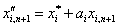, there,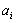is a constant of adjusting to make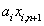to be a small amplitude chaos variable.

Step 6: Using the chaotic variables got by the second carrier, the iterated search would be continued, let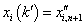, to calculate the corresponding function optimization value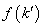, If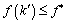then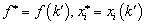; else, abandoned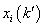. Let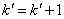. If the termination condition is satisfied, the optimal solution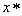is output; Otherwise, return to step 5, meanwhile, let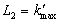, which is used as the number of fine search.

3. Determination of the Objective Function

The analytical solution to the well flow problem in the first type leakage aquifer is :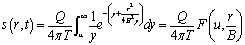(1)

There: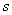is the aquifer drawdown, [L];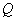is the pumping flow, [L3·T-1];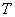is the hydraulic conductivity, [L2·T-1];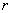is the distance between observation wells and pumping well, [L];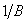is the leakage supply factor, [L-1].

The function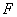was calculated in the method in literature , while the approximate method in literature [11, 12] is a corking way to calculate the part of Theis well flow in function. The pre-estimate parameters must be used to make the function of the formula (2) to achieve a minimum value when the chaotic time-series optimization algorithm was applied. That is to say the objective function is: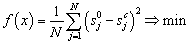(2)

There, the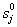is the observed drawdown at j-th moment, [L];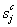is the calculated drawdown at j-th moment, [L];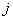is the parameters vector to be estimated;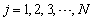is the serial number of the observation time of the drawdown during the pumping test.

4. Example

4.1. Data Source

We select three observation wells data in literature  to verify the application of chaotic time-series optimization algorithm in the first type leakage aquifer. Owing to the author selected the data which had a good correlation to calculated the parameters by Hantush approximate calculation method, namely, 17 observation data was chosen during 160-th min to 840-th min in observation well 1, 9 data during 227-th min to 900-th min in well 2, 7 data in well 3 during 363-th min to 850-th min. So we select the same data when we use the chaotic time-series optimization algorithm.

Table 1. Initial values of different parameters in different observation wells.

 Observation well Storage coefficient Hydraulic conductivity leakage factor Convergence value Rough search times Time-series length Rough search Fine search Well 1 0-0.015 0.4-1.99 15800-16000 0.0002251 0.000225 5 100 Well 2 0-0.015 0.4-2.11 15800-16000 0.000258 0.000257 20 400 Well 3 0-0.015 0.4-1.99 15800-16000 0.000051 0.00005 2 400

4.2. Results Contrast

The initial value of parameters of each observation wells in table 1 was introduced into the program, then the search results would be shown in table 2, the whole calculation process was accomplished with Visual Basic language.

Table 2. Comparison of results with different methods.

 Method Storage coefficient Hydraulic conductivity leakage factor Hantush approximate calculation method 0.000092 0.762 15900 Chaotic time-series optimization algorithm 0.000102 0.723 17557 Relative error (%) 10.9 5.1 10.4

As shown in Table 2, the calculated results was quite close between chaotic time-series optimization algorithm and Hantush approximate calculation method. The maximum relative error of the calculated results was 10.9%, the results of water conductivity are particularly accurate which relative error was 5.1%.

Compared the drawdown results calculated by chaotic time-series optimization algorithm whose parameters were based on table 2 with the actual observed values, in addition to some individual points relative error with large deviation, the remaining errors were all within ±2%. So the results obtained by the optimization algorithm of chaotic time-series were well simulated the drawdown of the three wells which was shown in figure 1.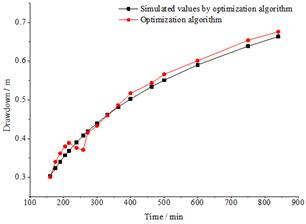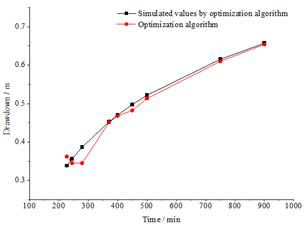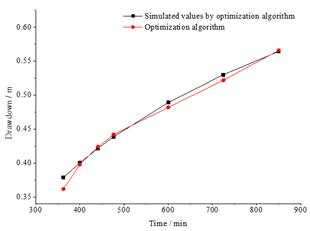Figure 1. The comparison between the results of Chaotic Time-Series Optimization Algorithm and actual values.

5. Control of Algorithm Parameters

5.1. Effect of Convergence Value

Through a lot of calculation results, it was found that the degree of the convergence value directly affects the times of coarse search and fine search in the program, especially for the rough search. With the first observation wells as the case, the relationship between the convergence value and the search times was shown in table 3 when the aquifer parameters were taken as the numerical values in table 1, the convergence of fine search was 0.0002251, the times of coarse search is limited to 100, the time-series length was 500.

Table 3. The relationship between convergence value and the times of search.

 Rough search convergence Rough search times Fine search times 0.00074 207 129 0.00064 177 105 0.00044 149 69 0.000074 102 9 0.000044 100 6

As shown in table 3, the convergence value of rough search has an osculating relationship with the times of rough search. When the convergence value of the coarse search was taken between 0.0005 and 0.00028, the times of coarse search had a little change, far less than the number of limited search, just changed from 1 to 19. However, when the convergence value of coarse search was close to the convergence of fine search, the times of rough search had a great change. This phenomenon was most obvious in the last two sets of data, the times of coarse search had changed from 60 to 100 while the convergence value of the coarse search just changed 0.000026899. In general, the times of rough search will increase with the decrease of convergence value. It's important to recognize that, this regularity was apparent when these two convergence values were close, but, when these two values were too close , the coarse will finish the search within the limited times, the times of rough search will have the same variation law with the convergence value. So, in the algorithm the difference between the two values cannot be too small.

5.2. Effect of Chaotic Time-Series Length

The length of chaotic time-series was achieved by Logistic iteration. The size of it actually reflects the degree of the state space. The length of the sequence was longer, the range of search was greater, the more full, and the more difficult to fall into local extremum. Theoretically speaking, the search accuracy would reach the highest when the time-series length was infinite. But in practical application it was bound to increase the search time, and also not realistic. To this end, we still use the observation well 1 as an example, the length of the chaotic time-series was selected from the 8 sets of data between 50 and 1000. The search results were shown in Table 4.

Table 4. The fine search times under different length of time-series and coarse search times.

 length of time-series coarse search times 2 5 10 20 30 50 100 50 5 7 4 3 4 5 8 100 5 9 7 7 4 5 5 200 6 2 5 2 4 4 6 400 4 2 2 2 2 4 3 500 4 2 2 2 2 5 3 600 4 2 2 2 2 4 3 800 3 2 2 2 2 4 3 1000 3 2 2 2 2 5 2

As can be seen in Table 4, under the same coarse search times, although the times of fine search was fluctuating, it still presented a clear downward trend with the increase of the length of chaotic time-series. This is due to the reason that the longer the length of the time-series was, the more fully the search was, and the results were more close to the real values of the parameters. However, the degree of the search had been sufficient when the length was over 400, and this change was no longer obvious. Combining with the results of the other two observation wells, the sequence length was limited between 100 and 600 was more suitable.

5.3. Control of Rough Search Times

The coarse search process was achieved by the iterative procedure of step 3. As mentioned above, when the length of chaotic time-series we got was long enough, the greater the times of coarse search was, the search in the state space was also more fully, and it was not easy to fall into local extremum. From table 4, with the increase of the times of rough search, the times of fine search will gradually became smaller and tend to be stable. In the rough search stage, we aimed to find the optimal solution of the problem, and ensure that the algorithm does not fall into the local extremum, so it will not require a large times of rough search, since such search in addition to increase computing time had not too much impact of fine search. So, for the algorithm in this paper, the times of coarse search between 2 and 20 was more appropriate.

5.4. Influence of Initial Parameters

The traditional gradient search algorithm used before would cause the search does not converge or the results were not unique when the initial value of the parameters to be estimated was not appropriate in solving the problem of nonlinear function optimization. In order to study the influence of initial value range of parameters to be estimated in chaos time-series optimization algorithm to search ability and search results, we first define the initial value of storage coefficient and leakage factor was consistent with the above, the minimum water conductivity was 0.4 and remain constant, the ceiling value was composed of 12 sets of data which was the numerical value between 2 and 5000 times of 1.99. The results of the calculation of fine search times and water conductivity were shown in Table 5 and table 6 respectively.

Table 5. The relationship between fine search times and the initial value of conductivity.

 Multiple of hydraulic conductivity T/times Rough search times: 2 Rough search times: 5 Time-series length: 200 Time-series length: 500 Time-series length: 200 Time-series length: 500 2 5 5 5 3 5 6 13 10 8 10 5 5 9 8 20 9 10 11 9 50 3 4 11 5 100 5 4 13 11 200 5 4 10 11 500 8 4 8 11 1000 8 4 10 6 2000 7 4 11 5 3000 7 5 10 5 5000 8 3 11 6

Table 5 shown that, no matter in what kind of combination of coarse search times and the time-series length, along with the increase of the hydraulic conductivity, although the number of the corresponding fine search times had some fluctuation there is a clear trend of increasing when the coefficient T of the water is 2 to 5000 times. When the coarse search number is 2 and the sequence length was 5, the times of fine search had no obvious change after the hydraulic conductivity was 50 times of 1.99. But to the general trend, fine search times increased with the increasing of hydraulic conductivity. On the other hand, under the same rough search times, the fine search times was smaller in the time-series length of 500 than that in 200. This also proved the applicability of the relationship between the fine search times and the length of chaotic time-series.

Table 6. The relationship between search results and the initial value of conductivity.

 Multiple of hydraulic conductivity T/times Rough search times: 2 Rough search times: 5 Time-series length: 200 Time-series length: 500 Time-series length: 200 Time-series length: 500 2 0.739 0.733 0.730 0.716 5 0.722 0.720 0.734 0.731 10 0.734 0.713 0.739 0.733 20 0.714 0.724 0.730 0.713 50 0.741 0.743 0.745 0.736 100 0.727 0.730 0.740 0.728 200 0.720 0.731 0.713 0.716 500 0.714 0.726 0.712 0.732 1000 0.729 0.719 0.736 0.732 2000 0.736 0.732 0.714 0.717 3000 0.713 0.714 0.728 0.724 5000 0.738 0.715 0.712 0.720

The data from table 6 shown that the phenomenon of no convergence was not appear in the search process of chaotic time-series optimization algorithm. Closed to the Hantush calculation result 0.76, the search results of the hydraulic conductivity were all between 0.712 and 0.745. The selection of initial parameters can affect the search speed of the algorithm, but it has no overt effect on its search ability and search results.

In addition the fluctuation of the search results of the leakage factor became large with the increasing of the initial value, which reduced the accuracy of the search results, the storage coefficient and the leakage factor also have the same conclusion as the hydraulic conductivity, after the same disposal method. The results of the other two observations wrer consistent with the results of the first observation well.

6. Conclusion

What conclusions we can got through the above analysis of the of chaotic time-series optimization algorithm and the calculation of the practical examples was that: chaotic time-series optimization algorithm can be effectively applied to the calculation problem of aquifer parameters; The difference of convergence value between rough search and fine search should be small enough and the closer the better; The length of chaotic sequences was suitable for 100~600 while the control of the times of rough search among 2~20; In view of the influence of the leakage factor searching results, the initial values of the parameters should be close to the reference values of the parameters to be estimated. In short, the chaotic time-series optimization algorithm was a new and effective method to analyze the pumping test and determine the parameters of the aquifer.

References

1. GUO Dong-ping, SONG Yan-xun, QIAN Hui. Groundwater dynamics [M]. Xi’an: Shanxi Science and Technique Publishing house, 1994.
2. WANG Yuan-hui, LIU Hui-yuan,GUO Jian-qing,et al. Estimation of anisotropic aquifer parameters based on chaos particle swarm algorithm[J].South-to-North Water Transfers and Water Science & Technology,2015,13(1):87-90.
3. SHI Zhi-yuan, XU Mo. Genetic algorithm inversion of the pumping test data to the hydrogeological parameters [J]. Sichuan Journal of Geology, 2011,31(4):466-469,480.
4. LUO Jun, LI Yan. Artificial bee colony algorithm with chaotic-search strategy [J]. Control and Decision, 2010,25(12): 1913-1916.
5. GUO Jianqing, LI Yan, WANG Hongsheng.et al. Chaotic time-Series optimization algorithm for aquifer parameter estimation [J]. China Rural Water and Hydropower, 2006, (12): 26-29.
6. Sushil, K Singh. Simple method for confined parameter estimation [J]. Journal of Irrigation and Drainage Engineering, 2000, 126(6): 404 – 407.
7. HU Xing-hua. Studies of chaotic optimization algorithm and its application [D]. Liaoning Technical University, 2008.
8. WANG Ling. Intelligent optimization algorithms with application [M]. Bing Jing: Tsinghua University press, 2001:105-106,108-110.
9. CHENG Chong-xi, LIN Min. Groundwater dynamics [M]. Wu Han: China University of Geosciences press, 1999.
10. YANG Tian-xing, FU Ze-zhou, LIU Jin-shan.et al. The principle and calculation method of unsteady flow of groundwater [M]. Bei Jing: Geological Publishing House, 1980.
11. ZHANG Juan-juan. Application of intelligent optimization algorithm in inversion of aquifer parameters [D]. Chang'an University.2006.
12. R. Srivastava. Implication of using approximation expressions for well function [J]. Journal of Irrigation and Drainage Engineering, 1995, 121(6):459-462.
13. Vedat Batu, Ph.D., P.E. Aquifer hydraulics: a comprehensive guide to hydrogeologic data [M]. JOHN WILEY & SONS, INC.1998:276-281.

 Contents 1. 2. 3. 4. 4.1. 4.2. 5. 5.1. 5.2. 5.3. 5.4. 6.
Article ToolsAbstractPDF(228K)